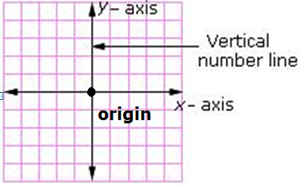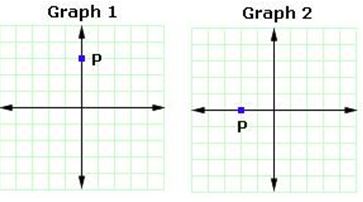## Definition Of Y-Axis

The Coordinate plane is formed by the intersection of two number lines, a horizontal number line and a vertical number line. The vertical number line is called the y-axis.

### Examples of Y Axis

The figure shows the coordinate plane formed by the intersection of the x and y-axes. Their point of intersection is called the origin### Solved Examples on Y-Axis

#### Ques: Which of the following graphs has a point P on the y-axis?A. Graph 1
B. Graph 2

#### Solution:

Step 1: The coordinate plane is formed by the intersection of two number lines, a horizontal number line and a vertical number line. The vertical number line is called the y-axis
Step 2: Graph 1 has the point P on the vertical number line i.e., on the y-axis.

A. (1, 0)
B. (-1, -1)
C. (0, 1)
D. (1, 1)